Gauge your potential with Aakash BYJU'S JEE Main'23 Mock Test Gauge your potential with Aakash BYJU'S JEE Main'23 Mock Test

# JEE Main 2021 Physics Paper With Solutions Feb 24 Shift 1

JEE Main 2021 Physics question paper for February 24, shift 1 exam is provided here. Students will also find solutions for each question covered in the Feb 24 JEE Main 2021 Physics shift 1 question paper. These solutions have been prepared by our subject experts and will help students to clearly understand the correct answer.

While referring to these JEE Main 2021 Physics questions and solutions students will be able to learn the problems quickly and easily. In essence, they will be able to prepare effectively for the upcoming exams. Students can access the solution videos along with JEE Main Physics question paper PDF for February 24, 2021, Shift 1 below.

## JEE Main 2021 24th February Shift 1 Physics Question Paper

JEE Main 2021 24th February Shift 1 Physics Question Paper

SECTION A

Question 1. Four identical particles of equal masses 1 kg made to move along the circumference of a circle of radius 1 m under the action of their own mutual gravitational attraction. The speed of each particle will be

a.

$$\begin{array}{l}\sqrt{\frac{(1+2\sqrt{2})G}{2}}\end{array}$$

b.
$$\begin{array}{l}\sqrt{G(1+2\sqrt{2})}\end{array}$$

c.
$$\begin{array}{l}\sqrt{\frac{G}{2}(2\sqrt{2}-1)}\end{array}$$

d.
$$\begin{array}{l}\sqrt{\frac{G}{2}(1+2\sqrt{2})}\end{array}$$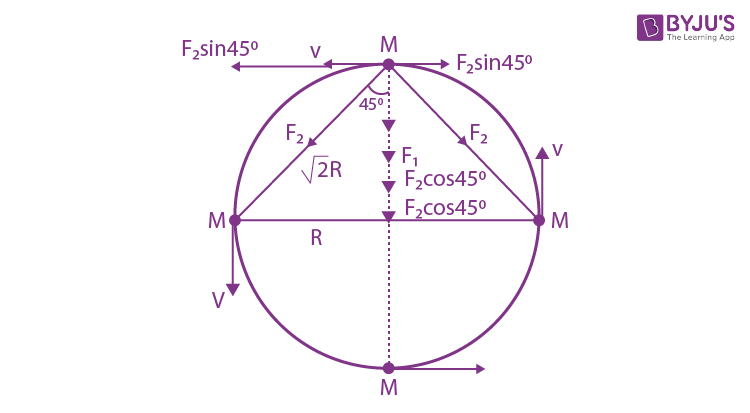⇒ By resolving force F2, we get

⇒ F1 + F2 cos 45° + F2 cos 45° = Fc

⇒ F1 + 2F2 cos 45° = Fc

Fc = centripetal force = MV2 / R

$$\begin{array}{l}\frac{GM^{2}}{(2R)^{2}} + \left [ \frac{2GM^{2}}{(2R)^{2}}cos 45^{o} \right ] = \frac{MV^{2}}{R}\end{array}$$

$$\begin{array}{l}\frac{GM^{2}}{4R^{2}} + \frac{2GM^{2}}{2\sqrt{2R^{2}}} = \frac{MV^{2}}{R}\end{array}$$

$$\begin{array}{l}\frac{GM}{4R} + \frac{GM}{\sqrt{2R}} = V^{2}\end{array}$$

$$\begin{array}{l}V = \sqrt{\frac{GM}{4R}+\frac{GM}{\sqrt{2.R}}}\end{array}$$

$$\begin{array}{l}V = \sqrt{\frac{GM}{R} \left [ \frac{1+2\sqrt{2}}{4} \right ]}\end{array}$$

$$\begin{array}{l}V = \frac{1}{2}\sqrt{\frac{GM}{R} (1+2\sqrt{2})}\end{array}$$

(Given: mass = 1 kg, radius = 1 m)

$$\begin{array}{l}V = \frac{1}{2}\sqrt{G(1+2\sqrt{2})}\end{array}$$

Question 2. Consider two satellites S1 and S2 with periods of revolution 1 hr and 8 hr respectively revolving around a planet in circular orbits. The ratio of angular velocity of satellite S1 to the angular velocity of satellite S2 is

a. 8: 1
b. 1: 8
c. 2: 1
d. 1: 4

We know that ω = 2𝜋/T

Given: Ratio of time period

T1 / T2 = 1/8

⇒ ω ∝ 1/T

⇒ ω1 / ω2 = T1 / T2

⇒ ω1 / ω2 = 8/1

⇒ω1: ω2 = 8 : 1

Question 3. n moles of a perfect gas undergoes a cyclic process ABCA (see figure) consisting of the following processes:

A→ B: Isothermal expansion at temperature T so that the volume is doubled from V1 to V2 and pressure changes from P1 to P2.

B → C: Isobaric compression at pressure P2 to initial volume V1.

C → A: Isochoric change leading to change of pressure from P2 to P1.

Total work done in the complete cycle ABCA is –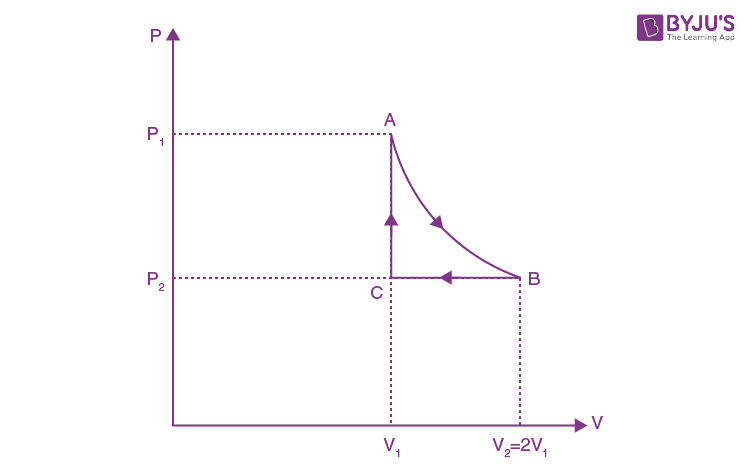a. 0
b. nRT(ln2 + 1/2)
c. nRTln2
d. nRT (ln2 – 1/2)A → B = isothermal process

B → C = isobaric process

C → A = isochoric process

Also, V2 = 2V1

Work done by gas in the complete cycle ABCA is –

⇒ w = wAB + wBC + wCA …. (1)

⇒ wCA = 0, as isochoric process

⇒ wAB = P1V1 ln (v2 / v1) = nRT ln ….(2)

⇒ wBC = P2 (V1 – V2) = P2 (V1 – 2V1) = -P2V1 = –nRT/2

⇒ Now putting the values of wAB, wBC and wCA in equation, we get

⇒ w = nRT ln (2) – nRT/2 + 0

⇒ w = nRT [ln (2) – 1/2]

⇒ w = nRT [ln (2) – 1/2]

Question 4. Two equal capacitors are first connected in series and then in parallel. The ratio of the equivalent capacities in the two cases will be

a. 2: 1
b. 1: 4
c. 4: 1
d. 1: 2

Given that first connection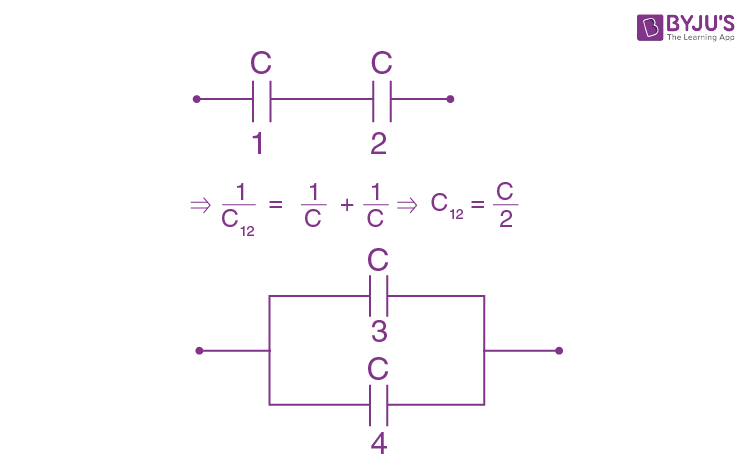Second connection

C34 = C + C = 2 C

Now, the ratio of equivalent capacities in the two cases will be –

⇒ C12 / C34 = C2 / 2C ⇒ C12 / C34 = ¼

Question 5. A cell E1 of emf 6V and internal resistance 2Ω is connected with another cell E2 of emf 4V and internal resistance 8Ω (as shown in the figure). The potential difference across points X and Y is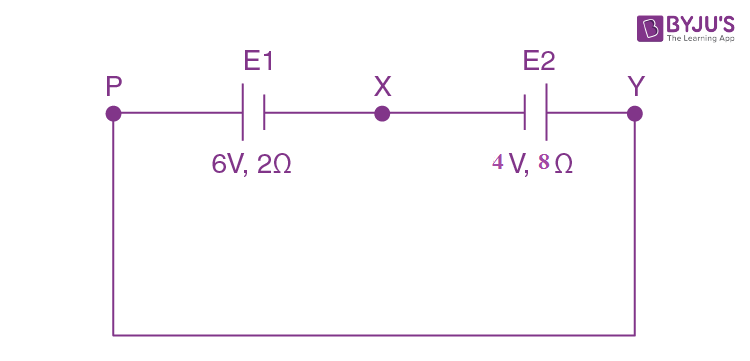a. 3.6V
b. 10.0V
c. 5.6V
d. 2.0V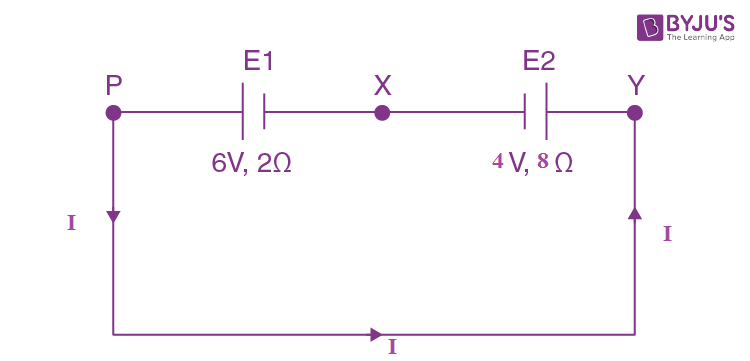emf of E1 = 6V

r1 = 2 Ω

emf of E2= 4V

r2 = 8Ω

|vx– vy| = potential difference across points X and Y

Eeff = 6 – 4 = 2V

Req = 2 + 8 = 10 Ω

So, current in the circuit will be

⇒ Ι = Eeff / Req⇒ I = 2/10 = 0.2 A

Now, potential difference across points X and Y is

|vx– vy| = E + iR

⇒ |vx– vy| = 4 + 0.2 × 8 = 5.6 V

⇒ |vx– vy| = 5.6 V

Question 6. If Y, K and η are the values of Young’s modulus, bulk modulus and modulus of rigidity of any material respectively. Choose the correct relation for these parameters.

a.

$$\begin{array}{l}k = \frac{Y\eta }{9\eta -3Y} N/m^{2}\end{array}$$

b.
$$\begin{array}{l}\eta = \frac{3YK}{9K + Y} N/m^{2}\end{array}$$

c.
$$\begin{array}{l}Y = \frac{9K\eta}{3K – \eta} N/m^{2}\end{array}$$

d.
$$\begin{array}{l}Y = \frac{9K\eta}{2\eta + 3K} N/m^{2}\end{array}$$

⇒ Y = 3K (1 – 2σ)

⇒ σ = ½ (1 – Y / 3K) …. (1)

⇒Y = 2η (1 + σ)

⇒ σ = Y / 2η – 1 …. (2)

By comparing equation (1) and (2), we get

⇒ Y / 2η – 1 = ½ (1 – Y / 3K)

⇒ Y / η – 2 = 1 – Y / 3K

⇒ Y / η = 1 + 2 – Y / 3K ⇒ Y / η = 3 – Y / 3K

⇒ Y / 3K = 3 – Y / η ⇒ Y / 3K = 3η – Y / η

⇒ K = ηY / 9η – 3Y

Question 7. Two stars of masses m and 2m at a distance d rotate about their common centre of mass in free space. The period of revolution is

a.

$$\begin{array}{l}2\pi = \sqrt{\frac{d^{3}}{3Gm}}\end{array}$$

b.
$$\begin{array}{l}\frac{1}{2\pi} = \sqrt{\frac{3Gm}{d^{3}}}\end{array}$$

c.
$$\begin{array}{l}\frac{1}{2\pi} = \sqrt{\frac{d^{3}}{3Gm}}\end{array}$$

d.
$$\begin{array}{l}2\pi = \sqrt{\frac{3Gm}{d^{3}}}\end{array}$$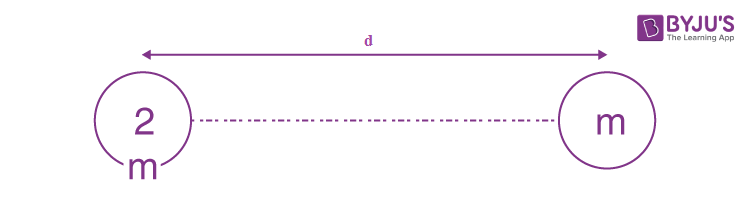⇒ G(m)(2m) / d2 = mω2 × 2d / 3

⇒ 2Gm / d2 = ω2 × 2d / 3

⇒ ω2 = 3Gm / d3

$$\begin{array}{l}\omega = \sqrt{\frac{3Gm}{d^{^{3}}}}\end{array}$$

We know that, ω = 2π / T so T = 2π / ω

$$\begin{array}{l}T = \frac{2\pi }{\sqrt{\frac{3Gm}{d^{3}}}} \Rightarrow T = 2\pi\sqrt{\frac{d^{3}}{3Gm}}\end{array}$$

Question 8. If the velocity-time graph has the shape AMB, what would be the shape of the corresponding acceleration-time graph?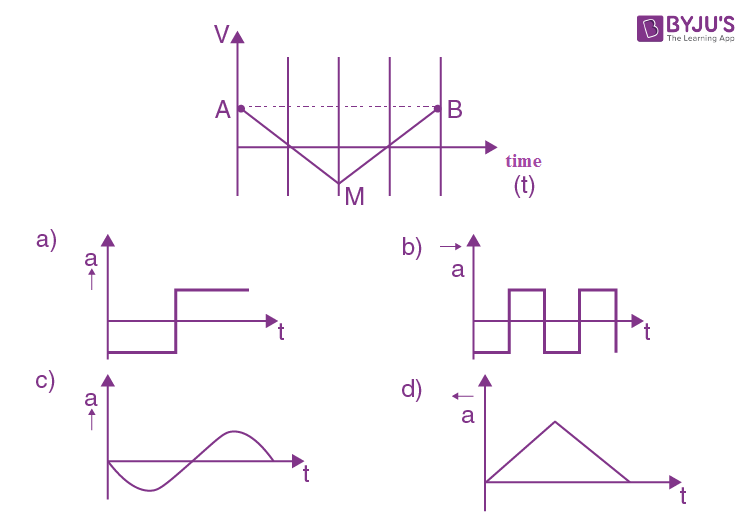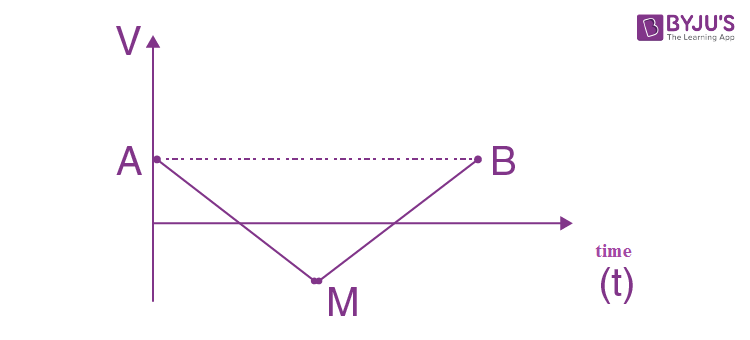a= dv / d t= slope of (v – t) curve

If m = +ve, then equation of straight line is

y = mx + c ⇒ v = mt + c (for MB)

If m = –ve, then equation of straight line is

y = –mx + c ⇒ v = -mt + c (for AM)

If we differentiate equation (1) and (2), we get

MB = +ve = m where m is constant.

AM = –ve = –m, so graph of (a-t) will be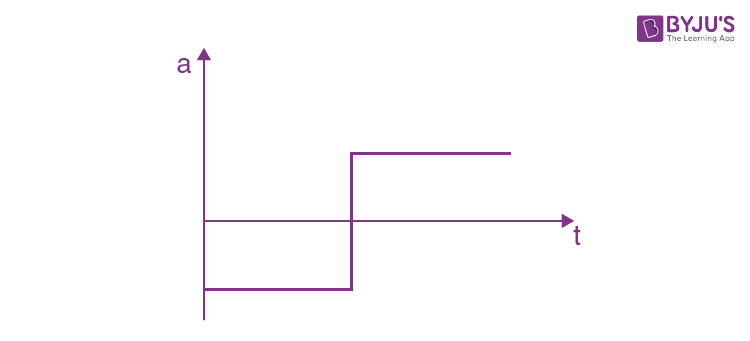Question 9. Given below are two statements:

Statement-I: Two photons having equal linear momenta have equal wavelengths.

Statement-II: If the wavelength of a photon is decreased, then the momentum and energy of a photon will also decrease.

In the light of the above statements, choose the correct answer from the options given below.

a. Statement-I is false but Statement-II is true
b. Both Statement-I and Statement-II are true
c. Both Statement-I and Statement-II are false
d. Statement-I is true but Statement-II is false

Energy of photon

E = hc / λ

= E ∝ 1 / λ

Hence, energy of photon increases as the wavelength of light decreases. Also, momentum of the photon p = E / c

⇒ p ∝ E

Thus, momentum of the photon also increases as its wavelength decreases.

Question 10. A current through a wire depends on time as i = α0t + βt2 where α0 = 20 A/s and β = 8 As-2. Find the charge crossed through a section of the wire in 15 s.

a. 2100 C
b. 260 C
c. 2250 C
d. 11250 C

Given: i = α0t + βt2

α = 20 A/s and β = 8As-2

t = 15 sec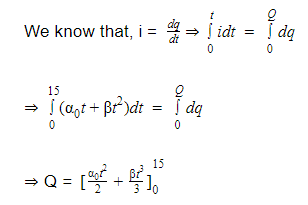⇒ Q = 20×15×15 / 2 + 8×15×15×15 / 3 – 0

⇒ Q = 11250 C

Question 11. Match List-I with List-II

 List – I List – II (a) Isothermal (i) Pressure constant (b) Isochoric (ii) Temperature constant (c) Adiabatic (iii) Volume constant (d) Isobaric (iv) Heat content is constant

Choose the correct answer from the options given below:

a. (a) – (ii), (b) – (iv), (c) – (iii), (d) – (i)
b. (a) – (ii), (b) – (iii), (c) – (iv), (d) – (i)
c. (a) – (i), (b) – (iii), (c) – (ii), (d) – (iv)
d. (a) – (iii), (b) – (ii), (c) – (i), (d) – (iv)

(a)→ (ii), (b)→ (iii), (c)→ (iv), (d)→ (i)

By theory,

In an isothermal process, temperature is constant.

In an isochoric process, volume is constant.

In an adiabatic process, heat content is constant.

In an isobaric process, pressure is constant.

Question 12. In the given figure, the energy levels of hydrogen atom have been shown along with some transitions marked A, B, C, D and E.

The transition A, B and C respectively represents: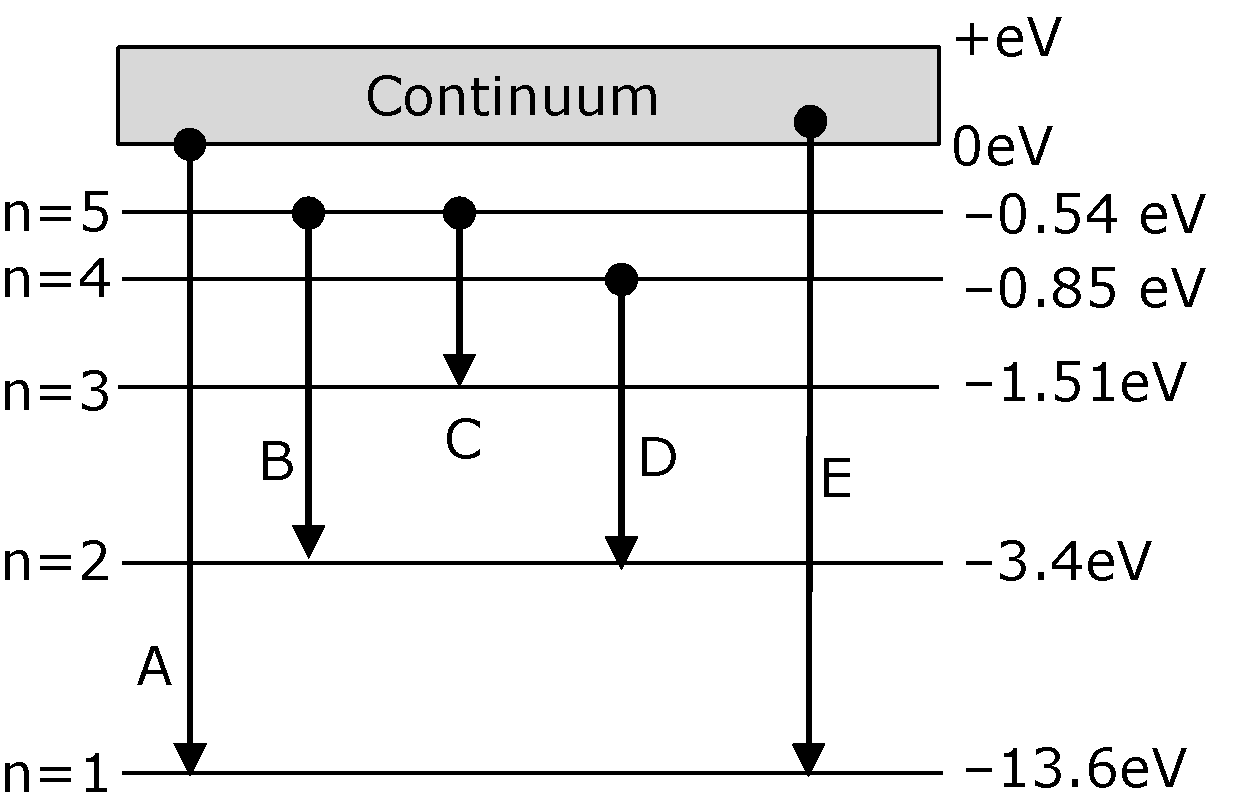a. The series limit of Lyman series, third member of Balmer series and second member of Paschen series
b. The first member of the Lyman series, third member of Balmer series and second member of Paschen series
c. The ionization potential of hydrogen, second member of Balmer series and third member of Paschen series
d. The series limit of Lyman series, second member of Balmer series and second member of Paschen series

A → series limit of Lyman series.

B → 3rd member of Balmer series.

C → 2nd member of Paschen series.

Question 13. The focal length f is related to the radius of curvature r of the spherical convex mirror by:

a. f = r
b. f = – ½ r
c. f = +½ r
d. f = – r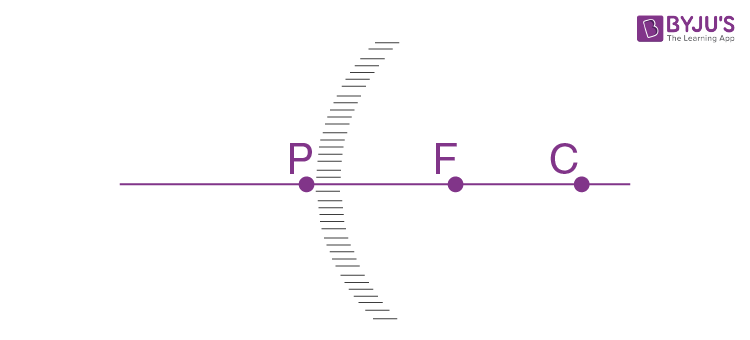So, r / 2 = f

f = +½ r

Question 14. Moment of inertia (M.I.) of four bodies, having same mass and radius, are reported as:

I1 = M.I. of thin circular ring about its diameter,

I2 = M.I. of circular disc about an axis perpendicular to disc and going through the centre,

I3 = M.I. of solid cylinder about its axis and

I4 = M.I. of solid sphere about its diameter.

Then:

a. I1 = I2 = I3< I4
b. I1 + I2 = I3 + 5/2 I4
c. I1 + I3< I2 + I4
d. I1 = I2 = I3> I4

Given,

I1 = M.I. of thin circular ring about its diameter

I2 = M.I. circular disc about an axis perpendicular to disc and going through the centre.

I3 = M.I. of solid cylinder about its axis

I4 = M.I. of solid sphere about its diameter

We know that,

I1= MR2 / 2

I2 = MR2 / 2

I3 = MR2 / 2

I4 = ⅖ MR

So, I1 = I2 = I3 > I4

Question 15. The work done by a gas molecule in an isolated system is given by,

$$\begin{array}{l}\alpha \cdot \beta ^{2}\cdot e^{-\frac{x^{2}}{\alpha .KT}}\end{array}$$
, where x is the displacement, k is the Boltzmann constant and T is the temperature.α and β are constants. Then the dimensions of β will be:

a. [M0LT0]
b. [M2LT2]
c. [MLT–2]
d. [ML2T–2]

Given: work done =

$$\begin{array}{l}\alpha \cdot \beta ^{2}\cdot e^{-\frac{x^{2}}{\alpha .KT}}\end{array}$$

k = boltzmann constant

T = temperature

x = displacement

We know that, x2 / α.k.T = dimensionless

[x2 / α.k.T ] = [M0L0T0] [α] = [L2 / k.T]

⇒ [κ] = [M1L2T-2K-1] [T] = [K]

⇒ [α] = [L2 / M1L2T-2K-1× K] ⇒ [α] = [M-1T2]

⇒ ω = α.β2

⇒ [M1L1T-2][L-1] / [M-1T2] = [β2] = [M2L2T-4] [β] = [MLT-2]

Question 16. If an emitter current is changed by 4mA, the collector current changes by 3.5 mA. The value of β will be:

a. 7
b. 0.875
c. 0.5
d. 3.5

Given:

ΔIE = 4 mA

ΔIC = 3.5 mA

We know that, α = ΔIC / ΔIE

⇒ α = 3.5 / 4 = ⅞

Also, β = α / 1-α, so

$$\begin{array}{l}\beta = \frac{\frac{7}{8}}{1-\frac{7}{8}} = \frac{7}{1}\end{array}$$

β = 7

Question 17. In Young’s double-slit experiment, the width of one of the slits is three times the other slit. The amplitude of the light coming from a slit is proportional to the slit-width. Find the ratio of the maximum to the minimum intensity in the interference pattern.

a. 4: 1
b. 2: 1
c. 3: 1
d. 1: 4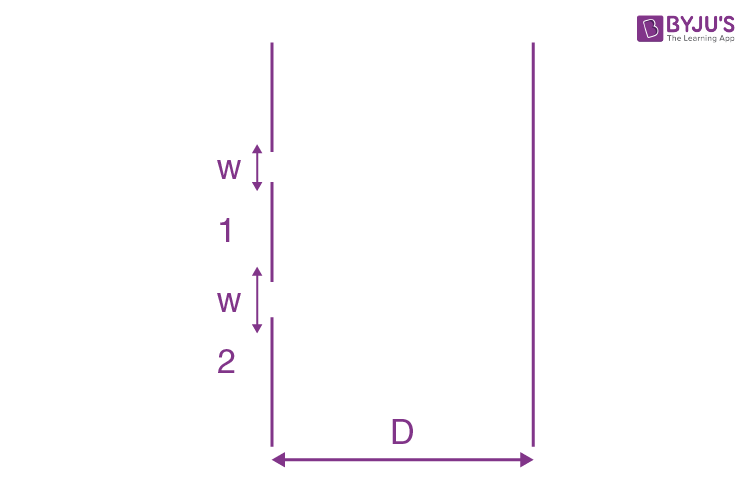Given: w2 = 3w1

Also, A ∝ w

w1 / w2 = ⅓ … (1)

Assume w1 = x, w2 = 3x

We know that

Imax = (A1 + A2)2, and

Imin = (A1 – A2)2

A1 / A2 = w1 / w2 ….(2)

From equation (2) we can say that

A1 = A and A2 = 3A

Now,

$$\begin{array}{l}\frac{I_{max}}{I_{min}} = \frac{(A+3A)^{2}}{(A – 3A)^{2}} = \frac{16A^{2}}{4A^{2}} = \frac{4}{1}\end{array}$$

$$\begin{array}{l}\frac{I_{max}}{I_{min}} = \frac{4}{1}\end{array}$$

Question 18. In the given figure, a mass M is attached to a horizontal spring which is fixed on one side to a rigid support. The spring constant of the spring is k. The mass oscillates on a frictionless surface with time period T and amplitude A. When the mass is in equilibrium position, as shown in the figure, another mass m is gently fixed upon it. The new amplitude of oscillation will be:a.

$$\begin{array}{l}A\sqrt{\frac{M}{M+m}}\end{array}$$

b.
$$\begin{array}{l}A\sqrt{\frac{M}{M-m}}\end{array}$$

c.
$$\begin{array}{l}A\sqrt{\frac{M-m}{M}}\end{array}$$

d.
$$\begin{array}{l}A\sqrt{\frac{M+m}{M}}\end{array}$$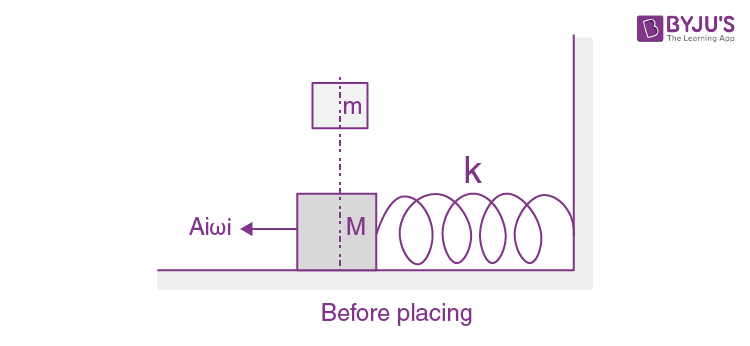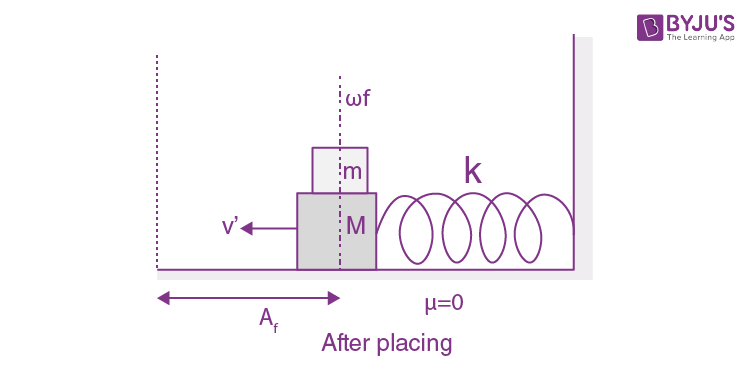We know that ωf =

$$\begin{array}{l}\sqrt{\frac{k}{M-m}}\end{array}$$
and ωi =
$$\begin{array}{l}\sqrt{\frac{k}{M}}\end{array}$$
Ai = A

Also, momentum is conserved just before and just after the block of mass (m) is placed because there is no impulsive force. So

MAiωi = (M + m) v’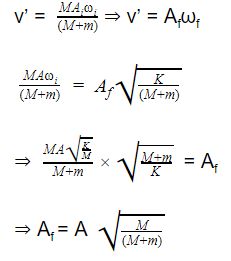Question 19. A cube of side ‘a’ has point charges +Q located at each of its vertices except at the origin where the charge is –Q. The electric field at the centre of cube is: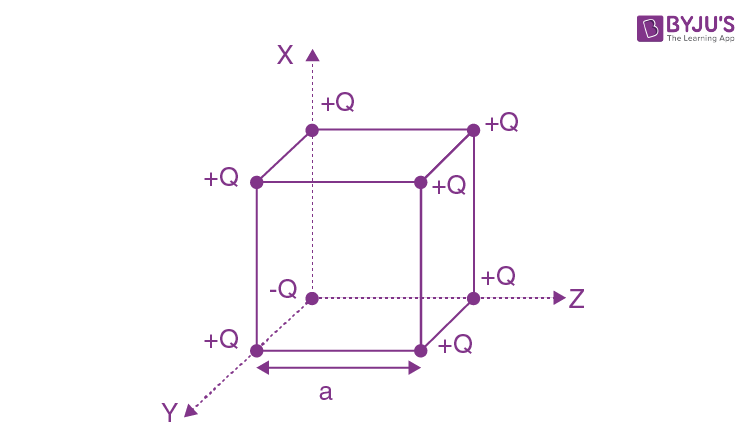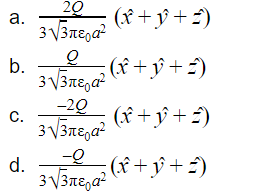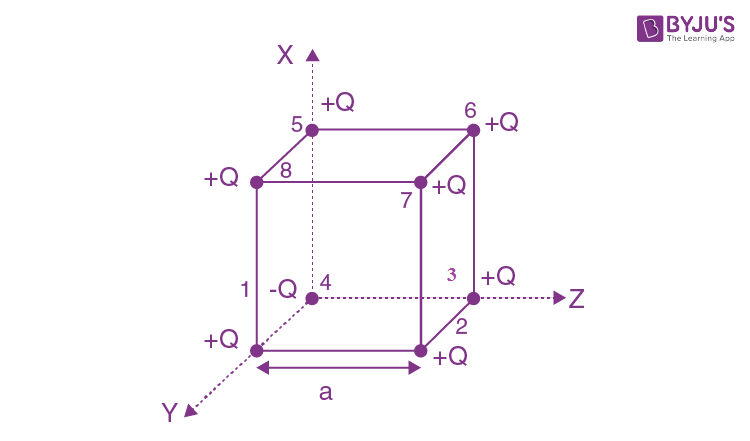We can replace –Q charge at origin by +Q and –2Q. Now due to +Q charge at every corner of the cube, the electric field at the centre of the cube is zero so now the net electric field at centre is only due to –2Q charge at origin.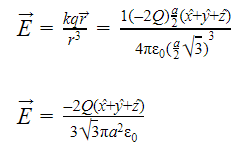Question 20. Each side of a box made of metal sheet in cubic shape is ‘a’ at room temperature ‘T’, the coefficient of linear expansion of the metal sheet is ‘α’. The metal sheet is heated uniformly, by a small temperature ΔT, so that its new temperature is T+ΔT. Calculate the increase in the volume of the metal box:

a. 4/3 πa3αΔT
b. 4πa3αΔT
c. 3a3αΔT
d. 4a3αΔT

Coefficient of volumetric expansion, γ = 3α

ΔV / V = γΔT

ΔV = V.γΔT

ΔV = a3. 3αΔT

SECTION-B

Question 1. A resonance circuit having inductance and resistance 2 × 10–4 H and 6.28 Ω respectively oscillates at 10 MHz frequency. The value of the quality factor of this resonator is________. [π = 3.14]

Given: R = 6.28 Ω

f = 10 MHz

L = 2 × 10-4 Henry

We know that quality factor Q is given by

⇒ Q = XL / R = ωL / R

also, ω = 2πf, so

⇒ Q = 2πfL / R

⇒ Q = 2π × 10 × 106 × 2 × 10-4 / 6.28 = 2000

Q = 2000

Question 2. A ball with a speed of 9 m/s collides with another identical ball at rest. After the collision, the direction of each ball makes an angle of 30° with the original direction. The ratio of velocities of the balls after collision is x : y, where x is _________.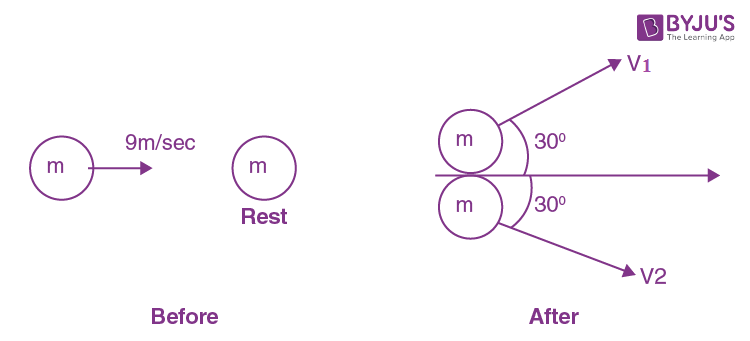Momentum is conserved just before and just after the collision in both x-y direction.

In y-direction,

pi = 0

pf = mv1 sin30o – mv2 sin30o

Pf = m × ½ v1 – m × ½ v2

pi = pf, so

= mv1 / 2 – mv2 / 2 = 0

⇒ mv1 / 2 = mv2 / 2 ⇒ v1 = v2

v1 / v2 = 1

Question 3. An audio signal υm = 20sin2π(1500t) amplitude modulates a carrier υc =80 sin 2π (100,000t). The value of percent modulation is ________.

We know that, modulation index = Am / Ac

From given equations, Am = 20 and Ac = 80

Percentage modulation index = Am / Ac × 100

⇒ 20 / 80 × 100 = 25%

The value of percentage modulation index is

= 25

Question 4. The coefficient of static friction between a wooden block of mass 0.5 kg and a vertical rough wall is 0.2. The magnitude of horizontal force that should be applied on the block to keep it adhere to the wall will be ______ N. [g = 10 ms-2]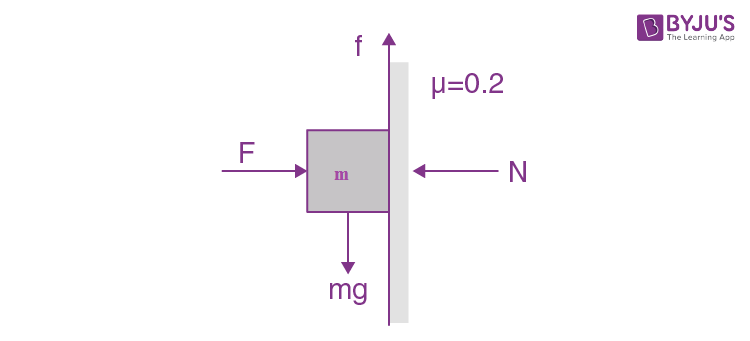Given: μs = 0.2

m = 0.5 kg

g = 10 m/s2

We know that

fs = μsN and …. (1)

To keep the block adhere to the wall

Here, N = F … (2)

fs = mg …. (3)

From equation (1), (2), and (3), we get

⇒mg = μs F

⇒ F = mg / μs ⇒ F = 0.5 × 10 / 0.2

F = 25 N (for all values of F greater than or equal to 25 N this case is possible)

Question 5. An inclined plane is bent in such a way that the vertical cross-section is given by y = x2 / 4 where y is in vertical and x in horizontal direction. If the upper surface of this curved plane is rough with a coefficient of friction μ = 0.5, the maximum height in cm at which a stationary block will not slip downward is _____ cm.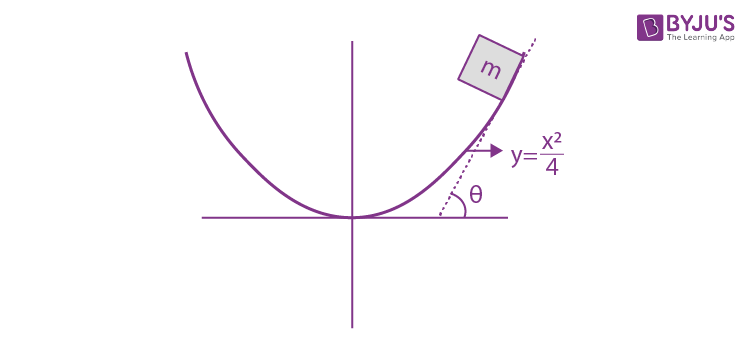Given,

y = x2 / 4

μ = 0.5

Condition for block will not slip downward

mg sin θ = μmg cos θ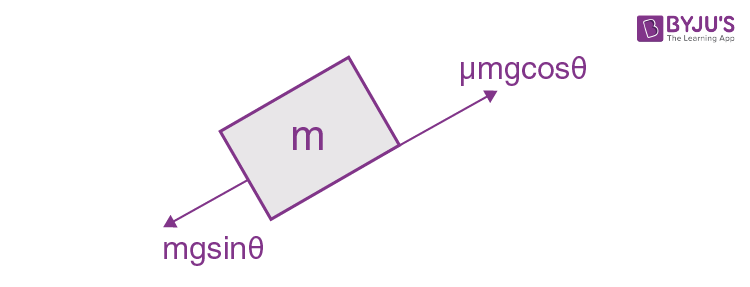⇒ tan θ = μ

And we know that

⇒ tanθ = dv / dx

⇒ dv / dx = μ ⇒ x/2 = 0.5 [y = x2 / 4 dy / dx = x / 2]

⇒ x = 1,

put x = 1 in equation y = x2/4

⇒ y = (1)2 / 4 ⇒ y = ¼ ⇒ y = 0.25

y = 25 cm

Question 6. An electromagnetic wave of frequency 5 GHz, is travelling in a medium whose relative electric permittivity and relative magnetic permeability both are 2. Its velocity in this medium is _______ × 107 m/s.

Given: f = 5 GHz

εr =2

μr = 2

Velocity of wave ⇒ v = c / n ….(1)

Where, n = √μrεr and c = speed of light = 3 × 108 m/s

n = √2 × 2 = 2

put the value of n in we get

⇒ v = 3 × 108 / 2 = 15 × 107 m/s

⇒ X × 107 = 15 × 107

X = 15

Question 7. A hydraulic press can lift 100 kg when a mass ‘m’ is placed on the smaller piston. It can lift _______ kg when the diameter of the larger piston is increased by 4 times and that of the smaller piston is decreased by 4 times keeping the same mass ‘m’ on the smaller piston.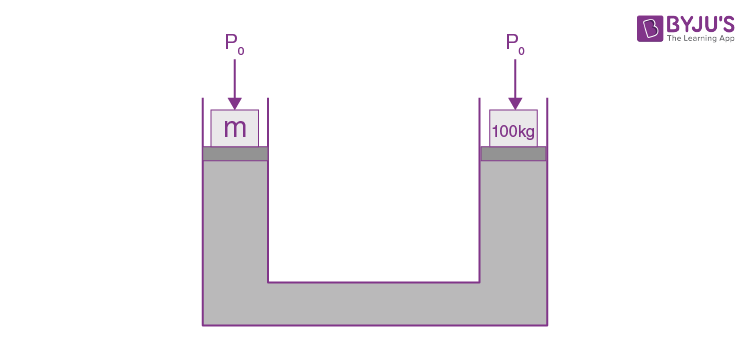Atmospheric pressure P0 will be acting on both the limbs of the hydraulic lift.

Applying pascal’s law for the same liquid level

⇒ P0 + mg / A1 = Po + (100)g / A2

⇒ mg / A1 = (100)g / A2 ⇒ m / 100 = A1/ A2 …(1)

Diameter of piston on side of 100 kg is increased by 4 times so new area = 16A2

Diameter of piston on side of (m) kg is decreasing

A1 = A1 / 16

(In order to increasing weight lifting capacity, diameter of smaller piston must be reduced)

Again, mg / (A1/16) = M’g / 16A2 ⇒ 256m / M’ = A1/ A2

From equation (1) = 256m / M’ = m / 100 ⇒ M’ = 25600 kg

Question 8. A common transistor radio set requires 12 V (D.C.) for its operation. The D.C. source is constructed by using a transformer and a rectifier circuit, which are operated at 220 V (A.C.) on standard domestic A.C. supply. The number of turns of the secondary coil are 24, then the number of turns of the primary are ______.

Given,

Primary voltage, Vp = 220 V

Secondary voltage, vs = 12 V

No. of turns in secondary coil is Ns = 24

No. of turns in primary coil, Np = ?

We know that for a transformer

⇒ Np / Ns = Vp / Vs

⇒ Np = Vp × Ns / Vs = 220 × 24 / 12

⇒ Np = 440

Question 9. An unpolarized light beam is incident on the polarizer of a polarization experiment and the intensity of the light beam emerging from the analyzer is measured as 100 Lumens. Now, if the analyzer is rotated around the horizontal axis (direction of light) by 30° in clockwise direction, the intensity of emerging light will be________ Lumens.

Given: I0 = 100 lumens, θ = 30o

Inet = I0 cos2θ

Inet = 100 × (√3/2)2 = 100 × 3 / 4

Inet = 75 lumens

Question 10. In connection with the circuit drawn below, the value of current flowing through the 2kΩ resistor is _______ × 10–4 A.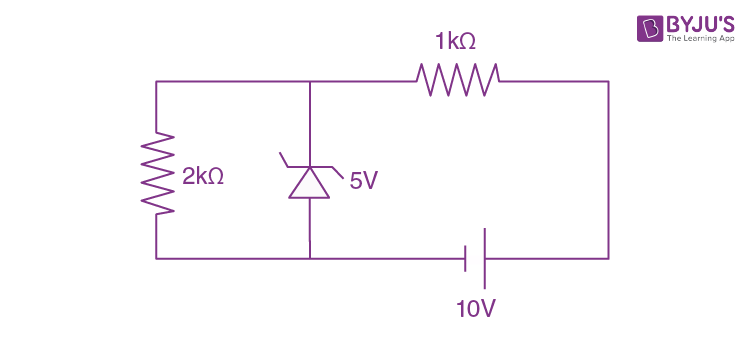In zener diode there will be o change in current after 5V

Zener diode breakdown

⇒ i = 5 / 2 × 103

⇒ i = 2.5 × 10–3 A

⇒ i = 25 × 10–4 A